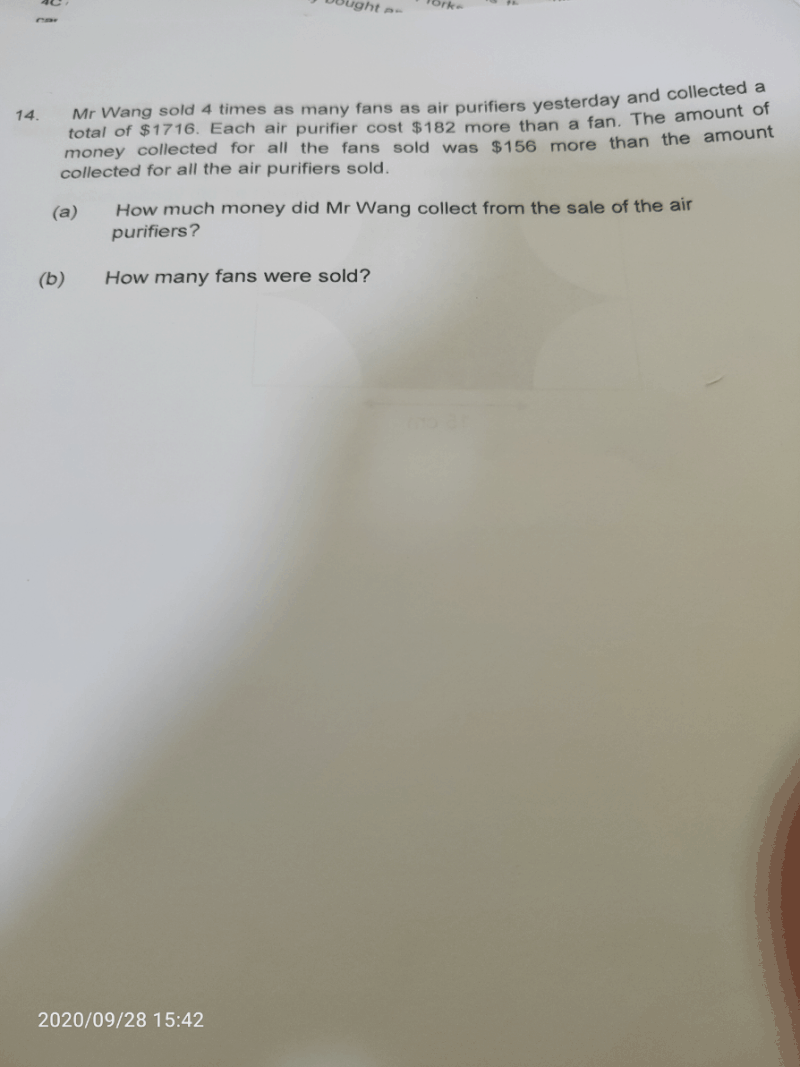# QuestionTotal cost of fans = (\$1716 + \$156) ÷ 2 = \$936

Total cost of air purifiers = \$1716 – \$936 = \$780 (a)

“There are 4 times as many fans as air purifiers.”

Let’s make the no. of air purifiers the same as fans,

Total cost of air purifiers = 4 x \$780 = \$3120

Diff in the total cost = \$3120 – \$936 = \$2184

Diff in individual cost = \$182

No. of fans = \$2184 ÷ \$182 = 12 (b)

Hope you understand and good luck with your exams! :))